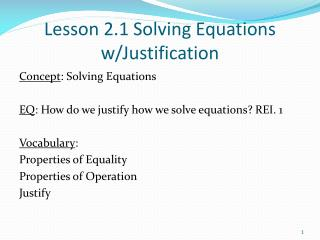DownloadDownload PresentationLesson 2.1 Solving Equations w/Justification

# Lesson 2.1 Solving Equations w/Justification

Download Presentation## Lesson 2.1 Solving Equations w/Justification

- - - - - - - - - - - - - - - - - - - - - - - - - - - E N D - - - - - - - - - - - - - - - - - - - - - - - - - - -
##### Presentation Transcript

1. Lesson 2.1 Solving Equations w/Justification Concept: Solving Equations EQ: How do we justify how we solve equations? REI. 1 Vocabulary: Properties of Equality Properties of Operation Justify

2. Solve the equations below, provide an explanation for your steps. • 2x – 3 = 13

3. Properties of Equality 2.1.1: Properties of Equality

4. Properties of Equality, continued 2.1.1: Properties of Equality

5. Properties of Equality, continued 2.1.1: Properties of Equality

6. Properties of Operations 2.1.1: Properties of Equality

7. Guided Practice Example 1 Which property of equality is missing in the steps to solve the equation –7x + 22 = 50? 2.1.1: Properties of Equality

8. Guided Practice: Example 1, continued Observe the differences between the original equation and the next equation in the sequence. What has changed? Notice that 22 has been taken away from both expressions, –7x + 22 and 50. 2.1.1: Properties of Equality

9. Guided Practice: Example 1, continued Refer to the table of Properties of Equality. The subtraction property of equality tells us that when we subtract a number from both sides of the equation, the expressions remain equal. The missing step is “Subtraction property of equality.” ✔ 2.1.1: Properties of Equality

10. Guided Practice: Example 1, continued 2.1.1: Properties of Equality

11. Guided Practice Example 2 Which property of equality is missing in the steps to solve the equation 2.1.1: Properties of Equality

12. Guided Practice: Example 2, continued Observe the differences between the original equation and the next equation in the sequence. What has changed? Notice that 3 has been added to both expressions, and 4. The result of this step is . 2.1.1: Properties of Equality

13. Guided Practice: Example 2, continued In order to move to the next step, the division of 6 has been undone. The inverse operationof the division of 6 is the multiplication of 6. The result of multiplying by 6 is –x and the result of multiplying 7 by 6 is 42. This matches the next step in the sequence. 2.1.1: Properties of Equality

14. Guided Practice: Example 2, continued Refer to the table of Properties of Equality. The multiplication property of equality tells us that when we multiply both sides of the equation by a number, the expressions remain equal. The missing step is “Multiplication property of equality.” ✔ 2.1.1: Properties of Equality

15. Guided Practice: Example 2, continued 2.1.1: Properties of Equality

16. Guided Practice: Example 3 What equation is missing based on the steps? Observe the 3rd and 5th equations. Read the 4th step. Fill in the missing equation. 2.1.1: Properties of Equality

17. You Try… Identify the property of equality that justifies each missing step or equation. 3. 4.

18. 5. Solve the equation that follows. Justify each step in your process using the properties of equality. Be sure to include the properties of operations, if used. 8(2x – 1) = 56

19. Summary… Identify the property represented below. x -3 = 6 x - 3 + 3 = 6 + 3 2. A = B, B = C, then A = C Solve the problem below justifying each step using the properties of equality. 3. 2x – 9 = 1

20. Solving Equations with the Variable in Both Expressions of the Equation Move the variable to solve for to the left of the equal sign. Move all other terms to the right of the equal sign. Combine like terms on each side of the equal sign. Now solve for the variable and simplify. Substitute the solution into the original equation and check your work.

21. Example 4: Solve the equation